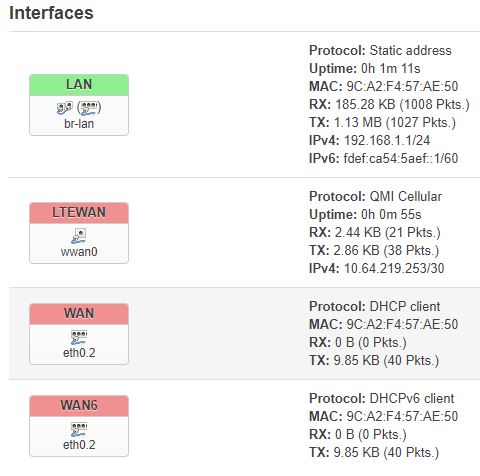# LTE not working with TL-MR6400 v5.3

I have installed openwrt to TL-MR6400 v5.3 with these instructions:

The installation goes ok but I can't access internet behind the WWAN from a LAN attached PC. The wwan gets ip address from ISP and shows it on the interface list as in the attached screenshot.I have tried deleting the WAN and WAN6 interfaces but that does not help.
LTEWAN is added to the firewall wan zone.
I have a prepaid SIM card which doesn't get public ip address.
Could this be the reason to the problem?
Or is there something different in the V5.3 device?
I also tried with version 22.03.3 of openwrt (see below) but it has same issue.
What could be wrong?
Thanks for any help!

### System

Hostname OpenWrt
Architecture MediaTek MT7628AN ver:1 eco:2
Target Platform ramips/mt76x8
Firmware Version OpenWrt 22.03.3 r20028-43d71ad93e / LuCI openwrt-22.03 branch git-22.361.69894-438c598

Can you access internet from the router itself?
Please show the output of the following commands (from the router):

``````ping 8.8.8.8
ip r
ip l
``````

No, this is what happens

## | |.-----.-----.-----.| | | |.----.| |_ | - || _ | -| || | | || || | |_____|| |||||___||| |____| || W I R E L E S S F R E E D O M

root@OpenWrt:~# ping 8.8.8.8
PING 8.8.8.8 (8.8.8.8): 56 data bytes
^C
--- 8.8.8.8 ping statistics ---
12 packets transmitted, 0 packets received, 100% packet loss
root@OpenWrt:~# ip r
default via 10.64.219.254 dev wwan0
10.64.219.252/30 dev wwan0 scope link src 10.64.219.253
192.168.1.0/24 dev br-lan scope link src 192.168.1.1
root@OpenWrt:~# ip l
1: lo: <LOOPBACK,UP,LOWER_UP> mtu 65536 qdisc noqueue state UNKNOWN qlen 1000
2: eth0: <BROADCAST,MULTICAST,UP,LOWER_UP> mtu 1500 qdisc fq_codel state UP qlen 1000
4: wlan0: <BROADCAST,MULTICAST> mtu 1500 qdisc noop state DOWN qlen 1000
5: wwan0: <POINTOPOINT,MULTICAST,NOARP,UP,LOWER_UP> mtu 1500 qdisc fq_codel state UNKNOWN qlen 1000
6: br-lan: <BROADCAST,MULTICAST,UP,LOWER_UP> mtu 1500 qdisc noqueue state UP qlen 1000
7: eth0.1@eth0: <BROADCAST,MULTICAST,UP,LOWER_UP> mtu 1500 qdisc noqueue master br-lan state UP qlen 1000
8: eth0.2@eth0: <BROADCAST,MULTICAST,UP,LOWER_UP> mtu 1500 qdisc noqueue state UP qlen 1000
root@OpenWrt:~#

`cat /sys/class/net/wwan0/qmi/raw_ip`
and
`cat /sys/kernel/debug/usb/devices`

Please use forum formatting when posting command output - look for `</>` icon.

``````
root@OpenWrt:~# cat /sys/class/net/wwan0/qmi/raw_ip
Y
root@OpenWrt:~#
root@OpenWrt:~# cat /sys/kernel/debug/usb/devices

T:  Bus=01 Lev=00 Prnt=00 Port=00 Cnt=00 Dev#=  1 Spd=480  MxCh= 1
B:  Alloc=  0/800 us ( 0%), #Int=  0, #Iso=  0
D:  Ver= 2.00 Cls=09(hub  ) Sub=00 Prot=00 MxPS=64 #Cfgs=  1
P:  Vendor=1d6b ProdID=0002 Rev= 5.10
S:  Manufacturer=Linux 5.10.161 ehci_hcd
S:  Product=EHCI Host Controller
S:  SerialNumber=101c0000.ehci
C:* #Ifs= 1 Cfg#= 1 Atr=e0 MxPwr=  0mA
I:* If#= 0 Alt= 0 #EPs= 1 Cls=09(hub  ) Sub=00 Prot=00 Driver=hub
E:  Ad=81(I) Atr=03(Int.) MxPS=   4 Ivl=256ms

T:  Bus=01 Lev=01 Prnt=01 Port=00 Cnt=01 Dev#=  2 Spd=480  MxCh= 0
D:  Ver= 2.00 Cls=00(>ifc ) Sub=00 Prot=00 MxPS=64 #Cfgs=  1
P:  Vendor=05c6 ProdID=9025 Rev= 3.18
S:  Manufacturer=Android
S:  Product=Android
S:  SerialNumber=91ec92ba
C:* #Ifs= 9 Cfg#= 1 Atr=a0 MxPwr=500mA
A:  FirstIf#= 6 IfCount= 3 Cls=01(audio) Sub=00 Prot=00
I:* If#= 0 Alt= 0 #EPs= 2 Cls=ff(vend.) Sub=ff Prot=ff Driver=(none)
E:  Ad=81(I) Atr=02(Bulk) MxPS= 512 Ivl=0ms
E:  Ad=01(O) Atr=02(Bulk) MxPS= 512 Ivl=0ms
I:* If#= 1 Alt= 0 #EPs= 2 Cls=ff(vend.) Sub=42 Prot=01 Driver=(none)
E:  Ad=02(O) Atr=02(Bulk) MxPS= 512 Ivl=0ms
E:  Ad=82(I) Atr=02(Bulk) MxPS= 512 Ivl=0ms
I:* If#= 2 Alt= 0 #EPs= 3 Cls=ff(vend.) Sub=00 Prot=00 Driver=(none)
E:  Ad=84(I) Atr=03(Int.) MxPS=  10 Ivl=32ms
E:  Ad=83(I) Atr=02(Bulk) MxPS= 512 Ivl=0ms
E:  Ad=03(O) Atr=02(Bulk) MxPS= 512 Ivl=0ms
I:* If#= 3 Alt= 0 #EPs= 3 Cls=ff(vend.) Sub=00 Prot=00 Driver=(none)
E:  Ad=86(I) Atr=03(Int.) MxPS=  10 Ivl=32ms
E:  Ad=85(I) Atr=02(Bulk) MxPS= 512 Ivl=0ms
E:  Ad=04(O) Atr=02(Bulk) MxPS= 512 Ivl=0ms
I:* If#= 4 Alt= 0 #EPs= 3 Cls=ff(vend.) Sub=ff Prot=ff Driver=qmi_wwan
E:  Ad=88(I) Atr=03(Int.) MxPS=   8 Ivl=32ms
E:  Ad=87(I) Atr=02(Bulk) MxPS= 512 Ivl=0ms
E:  Ad=05(O) Atr=02(Bulk) MxPS= 512 Ivl=0ms
I:* If#= 5 Alt= 0 #EPs= 2 Cls=08(stor.) Sub=06 Prot=50 Driver=(none)
E:  Ad=89(I) Atr=02(Bulk) MxPS= 512 Ivl=0ms
E:  Ad=06(O) Atr=02(Bulk) MxPS= 512 Ivl=125us
I:* If#= 6 Alt= 0 #EPs= 0 Cls=01(audio) Sub=01 Prot=00 Driver=(none)
I:* If#= 7 Alt= 0 #EPs= 0 Cls=01(audio) Sub=02 Prot=00 Driver=(none)
I:  If#= 7 Alt= 1 #EPs= 1 Cls=01(audio) Sub=02 Prot=00 Driver=(none)
E:  Ad=8a(I) Atr=05(Isoc) MxPS=  16 Ivl=1ms
I:* If#= 8 Alt= 0 #EPs= 0 Cls=01(audio) Sub=02 Prot=00 Driver=(none)
I:  If#= 8 Alt= 1 #EPs= 1 Cls=01(audio) Sub=02 Prot=00 Driver=(none)
E:  Ad=07(O) Atr=09(Isoc) MxPS=  16 Ivl=1ms

T:  Bus=02 Lev=00 Prnt=00 Port=00 Cnt=00 Dev#=  1 Spd=12   MxCh= 1
B:  Alloc=  0/900 us ( 0%), #Int=  0, #Iso=  0
D:  Ver= 1.10 Cls=09(hub  ) Sub=00 Prot=00 MxPS=64 #Cfgs=  1
P:  Vendor=1d6b ProdID=0001 Rev= 5.10
S:  Manufacturer=Linux 5.10.161 ohci_hcd
S:  Product=Generic Platform OHCI controller
S:  SerialNumber=101c1000.ohci
C:* #Ifs= 1 Cfg#= 1 Atr=e0 MxPwr=  0mA
I:* If#= 0 Alt= 0 #EPs= 1 Cls=09(hub  ) Sub=00 Prot=00 Driver=hub
E:  Ad=81(I) Atr=03(Int.) MxPS=   2 Ivl=255ms

``````

All looks good. Please install `tcpdump` package then open another connection to the router and run
`tcpdump -vv -i wwan0`
Then from the other connection run `ping 8.8.8.8` for a few seconds, then stop `tcpdump` with Ctrl-C and post its output.